# HSSlive: Plus One & Plus Two Notes & Solutions for Kerala State Board

## AP Board Class 7 Maths Chapter 9 Construction of Triangles Ex 3 Textbook Solutions PDF: Download Andhra Pradesh Board STD 7th Maths Chapter 9 Construction of Triangles Ex 3 Book AnswersAP Board Class 7 Maths Chapter 9 Construction of Triangles Ex 3 Textbook Solutions PDF: Download Andhra Pradesh Board STD 7th Maths Chapter 9 Construction of Triangles Ex 3 Book Answers

## Andhra Pradesh State Board Class 7th Maths Chapter 9 Construction of Triangles Ex 3 Books Solutions

 Board AP Board Materials Textbook Solutions/Guide Format DOC/PDF Class 7th Subject Maths Chapters Maths Chapter 9 Construction of Triangles Ex 3 Provider Hsslive

2. Click on the Andhra Pradesh Board Class 7th Maths Chapter 9 Construction of Triangles Ex 3 Answers.
3. Look for your Andhra Pradesh Board STD 7th Maths Chapter 9 Construction of Triangles Ex 3 Textbooks PDF.
4. Now download or read the Andhra Pradesh Board Class 7th Maths Chapter 9 Construction of Triangles Ex 3 Textbook Solutions for PDF Free.

## AP Board Class 7th Maths Chapter 9 Construction of Triangles Ex 3 Textbooks Solutions with Answer PDF Download

Find below the list of all AP Board Class 7th Maths Chapter 9 Construction of Triangles Ex 3 Textbook Solutions for PDF’s for you to download and prepare for the upcoming exams:

Question 1.
Construct Δ NET with measurement NE = 6.4 cm, ∠N = 50° and ∠E = 100°.
Solution:
NE = 6.4 cm, ∠N = 50° and ∠E = 100°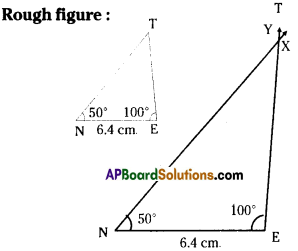Step -1: Draw a rough sketch of a triangle and label it with the given measurements.
Step -2: Draw a Line segment NE of length 6.4 cm.
Step -3: Draw a ray NX−→− maktng an angle 50° at N.
Step -4: Draw a ray EY−→− making an angle 100° at E. Extend the ray NX−→− if necessary to intersect ray EY−→−
Step – 5: Mark the intersecting point of the two rays as T.
We have the required ΔNET.

Question 2.
Construct ΔPQR such that QR =6 cm, ∠Q = ∠R = 60°. Measure the other two sides of the triangle and name the triangle.
Solution: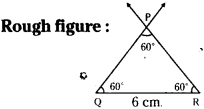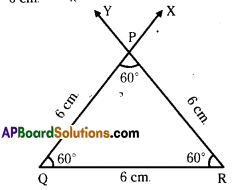QR = 6cm ∠Q = ∠R = 60°.
Step -1: Draw a rough sketch of a triangle and label it with the given measurements.
Step -2: Draw a line segment QR of length 6 cm.
Step -3: Draw a ray QX−→− making an angle 60° at Q
Step -4: Draw a ray RY−→− making an angle 60° at R.
Step -5: Mark the intersecting point of the two rays as P. PQ = 6cm and PR = 6 cm
∴ The triangle is an equilateral triangle.

Question 3.
Construct ΔRUN in which RN = 5cm, ∠R = ∠N = 45°. Measure the other angle and other sides. Name the triangle.
Solution:
RN = 5cm, ∠R = ∠N = 45°.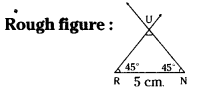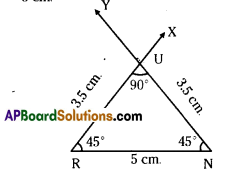Step -1: Draw a rough sketch of a triangle and label it with the given measurements.
Step -2: Draw a line segment RN of length 5cm.
Step -3: Draw a ray RX−→− making an angle 45° at R.
Step -4: Draw a ray NY−→− making an angle 45° at N.
Step -5: Mark the intersecting point of the two rays as U. ΔRUN is the required triangle
∠U = 90°, RU = UN = 3.5 cm.
∴ The triangle is an isosceles right angled triangle.

## Andhra Pradesh Board Class 7th Maths Chapter 9 Construction of Triangles Ex 3 Textbooks for Exam Preparations

Andhra Pradesh Board Class 7th Maths Chapter 9 Construction of Triangles Ex 3 Textbook Solutions can be of great help in your Andhra Pradesh Board Class 7th Maths Chapter 9 Construction of Triangles Ex 3 exam preparation. The AP Board STD 7th Maths Chapter 9 Construction of Triangles Ex 3 Textbooks study material, used with the English medium textbooks, can help you complete the entire Class 7th Maths Chapter 9 Construction of Triangles Ex 3 Books State Board syllabus with maximum efficiency.

## FAQs Regarding Andhra Pradesh Board Class 7th Maths Chapter 9 Construction of Triangles Ex 3 Textbook Solutions

#### Can we get a Andhra Pradesh State Board Book PDF for all Classes?

Yes you can get Andhra Pradesh Board Text Book PDF for all classes using the links provided in the above article.

## Important Terms

Andhra Pradesh Board Class 7th Maths Chapter 9 Construction of Triangles Ex 3, AP Board Class 7th Maths Chapter 9 Construction of Triangles Ex 3 Textbooks, Andhra Pradesh State Board Class 7th Maths Chapter 9 Construction of Triangles Ex 3, Andhra Pradesh State Board Class 7th Maths Chapter 9 Construction of Triangles Ex 3 Textbook solutions, AP Board Class 7th Maths Chapter 9 Construction of Triangles Ex 3 Textbooks Solutions, Andhra Pradesh Board STD 7th Maths Chapter 9 Construction of Triangles Ex 3, AP Board STD 7th Maths Chapter 9 Construction of Triangles Ex 3 Textbooks, Andhra Pradesh State Board STD 7th Maths Chapter 9 Construction of Triangles Ex 3, Andhra Pradesh State Board STD 7th Maths Chapter 9 Construction of Triangles Ex 3 Textbook solutions, AP Board STD 7th Maths Chapter 9 Construction of Triangles Ex 3 Textbooks Solutions,
Share: